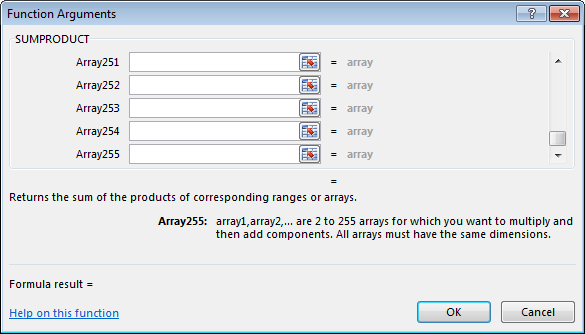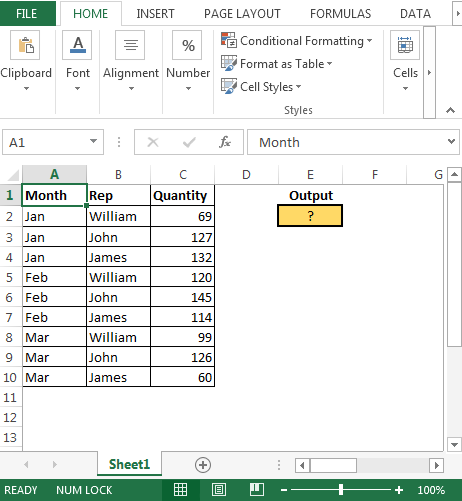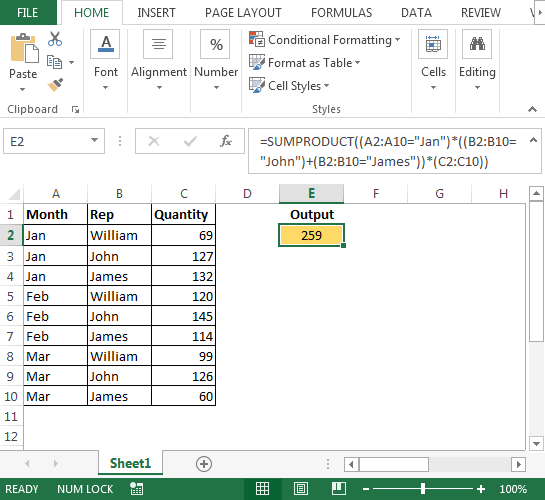SUMPRODUCT with Criteria in 2 Columns in Microsoft Excel 2010

In this article, we will focus on how to use SUMPRODUCT function with criteria in 2 columns & extract the output, meeting all the conditions.

Question):-

I have a three columns data comprising of Months in column A, Sales Rep in column B & Sales Quantity in column C. Now, I want to calculate the quantity sale by Rep John & James from January month.

SUMPRODUCT: Returns the sum of the products of corresponding ranges or arrays.

Syntax: =SUMPRODUCT(array1,array2,array3,…)

array1,array2,….. array255 are the ranges of cells or arrays that you want to multiply & then add all of them.

There can be maximum 255 arrays in Sumproduct function. Refer below screenshot:-Following is the snapshot of data we have:• In cell E2, the formula is
• =SUMPRODUCT((A2:A10=”Jan”)*((B2:B10=”John”)+(B2:B10=”James”))*(C2:C10))In case the criteria change as following:

• Month= Jan & Feb
• Rep=John & James

To get the output, we will use SUMPRODUCT function as follows:

• In Cell E2, the formula is
• =SUMPRODUCT(((A2:A10=”Jan”)+(A2:A10=”Feb”))*((B2:B10=”John”)+(B2:B10=”James”))*(C2:C10))

• The same result can be achieved through Filters but this requires user to first apply filter & then set criteria in Month & Rep column.
• Using SUMPRODUCT function we can avoid using filters in Excel.

In this way, we can use Sumproduct function to work with multiple conditions in two columns & update the quantity sale.Terms and Conditions of use

The applications/code on this site are distributed as is and without warranties or liability. In no event shall the owner of the copyrights, or the authors of the applications/code be liable for any loss of profit, any problems or any damage resulting from the use or evaluation of the applications/code.